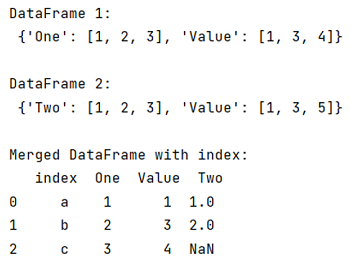# How to keep index when using pandas merge?

Learn to keep index when using pandas merge.
Submitted by Pranit Sharma, on June 11, 2022

DataFrames are 2-dimensional data structure in pandas. DataFrames consists of rows, columns and the data. The Data inside the DataFrame can be of any type.

## Problem statement

Sometimes, when we merge two DataFrames, the resulting DataFrame has some index pattern which is not our requirement. We want to set the index after merging according to our wish.

## Keep index when using pandas merge

To keep index when using pandas merge, first create two separate DataFrames, then reset the index using DataFrame.reset_index() method. As we reset the index, we will merge the DataFrame with another DataFrame also we will pass 'how = left' as a parameter if we want the indices of 1st DataFrame or 'how = right' if we want the indices of 2nd DataFrame.

To work with pandas, we need to import pandas package first, below is the syntax:

```import pandas as pd
```

Let us understand with the help of an example,

## Python program to keep index when using pandas merge

```# Importing pandas package
import pandas as pd

# Creating a dictionary
d1= {
'One': [ 1, 2, 3],
'Value': [ 1, 3, 4]
}

d2 = {
'Two': [1, 2, 3],
'Value': [1, 3, 5]
}

# Creating dataframes
df1 = pd.DataFrame(d1, index=['a','b','c'])
df2 = pd.DataFrame(d2, index=[0,1,2])

# Display both DataFrames
print("DataFrame 1:\n",d1,"\n")
print("DataFrame 2:\n",d2,"\n")

# Setting index
result = df1.reset_index().merge(df2,how='left')

# Display result
print("Merged DataFrame with index:\n",result)
```

## Output

The output of the above program is: